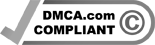#### Calculus (noun, “KALK-yoo-luss”)

Calculus is a type of math. Specifically, it is math that deals with change. It was invented in the 17th century by two separate thinkers. One was German mathematician Gottfried Leibniz. The other was the English physicist Isaac Newton.

There are two branches of calculus. The first is “differential” calculus. This math is used to determine how much something is changing at a given time or place. For instance, it can be used to find how much a curved line is pointing up or down at any spot along that line. The second branch is “integral” calculus. This math is used find quantities based on their rate of change. For instance, it can be used to find the area under a line whose curvature is known.

Say, for example, that you make a graph plotting a car’s speed over time. As the car drives, it changes its speed. It speeds up as it sets off down the road. And it slows down as it approaches a stoplight. When you plot the car’s changing speed, the line on your graph will wiggle up and down. Differential calculus will tell you how much that wiggling line is pointed up or down at any given spot. That is, it will tell you how much the car’s speed is changing (its acceleration) at any given point in time.

Integral calculus, meanwhile, will help you find the area under that wiggling line. And the area under a line plotting speed over time is equal to the total distance traveled. So, with calculus, you can use a plot of a car’s speed over time to find the total distance the car has driven.

Calculus is a powerful tool that can describe many things. The orbits of planets around the sun. The total pressure behind a dam where water is rising. How fast diseases spread. Calculus can be applied to most anything that is changing over space or time.

#### In a sentence

Calculus can be used to find the volume of even complexly shaped objects, such as icicles.

Check out the full list of Scientists Say.

Related Posts